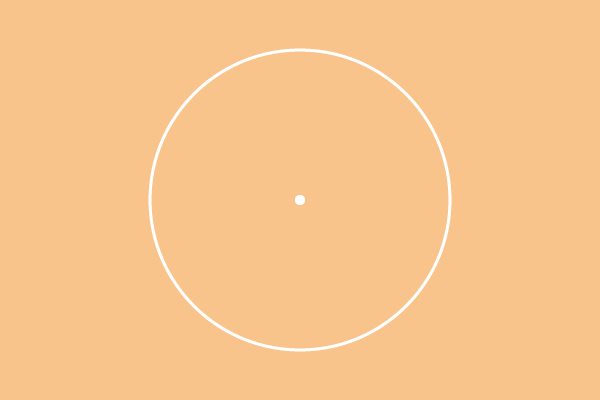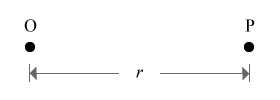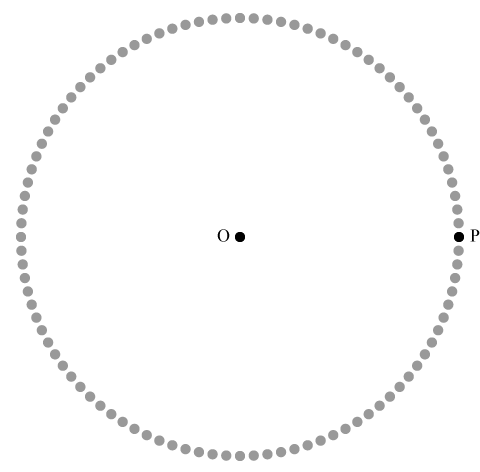# Circle

## Definition

The locus of a moving point that maintains equidistant from a fixed point is called a circle.Circle is a plane round shape figure and it is one of the most significant geometrical shapes in geometry. It is actually formed in two different ways.

1. A point which moves around another point with equidistant.
2. Joining the points which are located at equal distance from a point.

These two methods form round shape figure geometrically and it is called as circle. Here, you understand how exactly a circle is formed in geometric system.

## ConstructionConsider a point in the plane and it is called as point $O$.

Consider another point in the plane and assume to call it as point $P$ and it is located at $r$ units’ distance from the point $O$.

Identify the points which are located at equal distance ($r$ units) from the point $O$. Therefore, the distance from point $O$ to point $P$ is exactly equal to distance from point $O$ to each surrounded point displayed in the below figure.Now, join all the points and it forms a round shape figure, called circle.

Instead, assume the point $P$ is moved in round shape by maintaining equal distance from the point $O$. The point $P$ is known as variable point because it represents all the points which are located at same distance from point $O$. The locus of the moving point gives a round plane figure and it is known as circle. The locus of variable point can be understood in this video tutorial.

Latest Math Topics
Latest Math Problems
Email subscription
Math Doubts is a best place to learn mathematics and from basics to advanced scientific level for students, teachers and researchers. Know more